# Inverse Trigonometric Functions: Definition & Problems

Lesson Transcript
Instructor
Jennifer Beddoe

Jennifer has an MS in Chemistry and a BS in Biological Sciences.

Expert Contributor
Robert Ferdinand

Robert Ferdinand has taught university-level mathematics, statistics and computer science from freshmen to senior level. Robert has a PhD in Applied Mathematics.

In mathematics, an inverse operation can reverse the effect of another operation. This lesson explains how inverse trigonometric functions work and provides examples of how they are used in scientific fields and real-life situations. Updated: 05/10/2021

## What Are Inverse Trig Functions?

Every mathematical function, from the simplest to the most complex, has an inverse, or opposite. For addition, the inverse is subtraction. For multiplication, it's division. And for trigonometric functions, it's the inverse trigonometric functions.

Trigonometric functions are the functions of an angle. The term ''function'' is used to describe the relationship between two sets of numbers or variables. In modern mathematics, there are six basic trigonometric functions: sine, cosine, tangent, secant, cosecant, and cotangent. The inverse trigonometric functions are inverse sine, inverse cosine, inverse tangent, inverse secant, inverse cosecant, and inverse cotangent.

An error occurred trying to load this video.

Try refreshing the page, or contact customer support.

Coming up next: SohCahToa: Definition & Example Problems

### You're on a roll. Keep up the good work!

Replay
Your next lesson will play in 10 seconds
• 0:04 What Are Inverse Trig…
• 0:49 Trigonometric Ratios
• 2:14 Inverse Trig Functions
• 3:07 Example Problems
• 4:25 Lesson Summary
Save Save

Want to watch this again later?

Log in or sign up to add this lesson to a Custom Course.

Timeline
Autoplay
Autoplay
Speed Speed

## Trigonometric Ratios

The trigonometric functions can all be defined as ratios of the sides of a right triangle. Since all right triangles conform to the Pythagorean theorem, as long as the angles of two right triangles are the same, their sides will be proportional. Because of this, the ratios of one side to another will always be the same. Take a look at this example: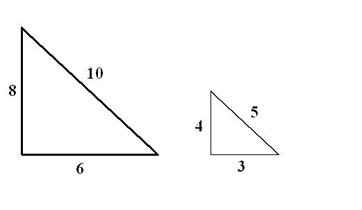These triangles have the same angle measures, so their sides are proportional. Any ratio of one side to another will be the same for both triangles.

6/10 = 3/5

By discovering that these ratios are the same for any sized right triangle (as long as they have the same angle measure), the trigonometric functions were discovered. These functions relate one angle of a triangle to the ratio of two of its sides: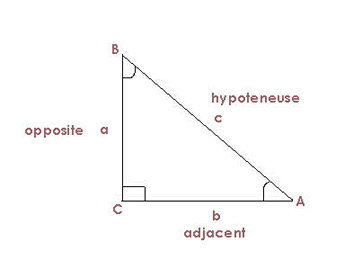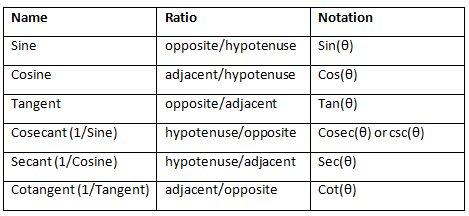Because of these ratios, when an angle (other than the right angle) of a right triangle and at least one side are known, you can determine the length of the other sides using these ratios. And, inversely, when the lengths of the two sides are known, the angle measure can be determined.

Since memorizing these ratios can prove to be difficult, there is a mnemonic that helps keep them straight. SOH CAH TOA is a helpful device to remember which ratio goes with which function:

Sine = Opposite / Hypotenuse

Cosine = Adjacent / Hypotenuse

Tangent = Opposite / Adjacent

## Inverse Trig Functions

The inverse trigonometric functions are used to determine the angle measure when at least two sides of a right triangle are known. The particular function that should be used depends on what two sides are known. For example, if you know the hypotenuse and the side opposite the angle in question, you could use the inverse sine function. If you know the side opposite and the side adjacent to the angle in question, the inverse tangent is the function you need.

To unlock this lesson you must be a Study.com Member.
Create your account

Additional Activities

## Practice Problems: Inverse Trigonometric Functions

#### Key Terms

• Inverse Trigonometric Functions: Functions that give the value of the angle in Degrees or Radians from its Trigonometric Function Value.

#### Materials Needed

• Paper
• Pencil
• Scientific Calculator in Degree Mode

#### Practice Problems: Show All Your Work

(a) Triangle ABC has a right angle at C. Next, side AB = 7 m and side CB = 4 m. Using inverse Trigonometric functions, find the degree measures of angle B and angle A.

(b) Triangle EFG has a right angle at G. Next, side EG = 3 in and side GF = 2 in. Using inverse Trigonometric functions, find the degree measures of angle E and angle F.

#### Answers (To Check Your Work):

(a) Below is a sketch of triangle ABC.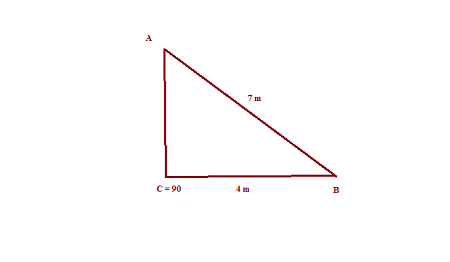Since ABC is a right-angled triangle with a right angle at C, we can use the Trigonometric Functions in a right triangle to get the ratio:

cos B = adjacent/hypotenuse = 4/7.

Applying the inverse cosine function to both sides of the above relation yields:

cos^(-1) (cos B) = cos^(-1) (4/7).

Using a scientific calculator in degree mode gives us:

B = 55.15 degrees.

Next, since the sum of the angles of a triangle is 180 degrees, we get angle A = 180 - (angle B + angle C) = 180 - (55.15 + 90) = 34.85 degrees.

Angle B = 55.15 degrees and angle A = 34.85 degrees.

(b) Below is a sketch of triangle EFG.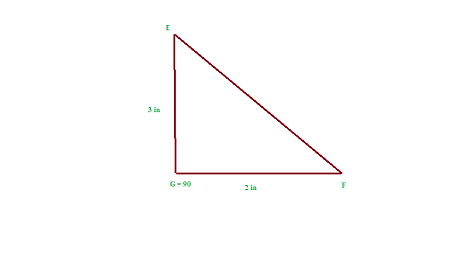Since EFG is a right-angled triangle with a right angle at G, we can use the Trigonometric Functions in a right triangle to get the ratio:

tan F = opposite/adjacent = 3/2.

Applying the inverse tangent function to both sides of the above relation yields:

tan^(-1) (tan F) = tan^(-1) (3/2).

Using a scientific calculator in degree mode gives us:

F = 56.31 degrees.

Next, since the sum of the angles of a triangle is 180 degrees, we get angle E = 180 - (angle F + angle G) = 180 - (56.31 + 90) = 33.69 degrees.

Angle F = 56.31 degrees and angle E = 33.69 degrees.

### Register to view this lesson

Are you a student or a teacher?

### Unlock Your Education

#### See for yourself why 30 million people use Study.com

##### Become a Study.com member and start learning now.
Back
What teachers are saying about Study.com
Create an account to start this course today
Used by over 30 million students worldwide